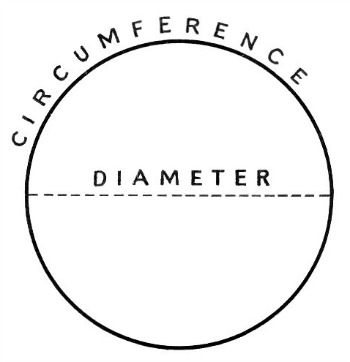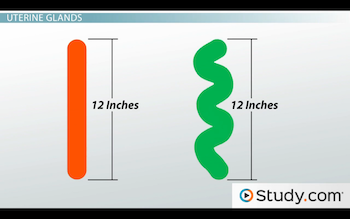# 4th Grade Math Vocabulary: Lesson for Kids

Instructor: Cori Hodges

Cori has degrees in Elementary Education and Early Childhood Education and has taught lower elementary.

This lesson contains some of the words that are often used in 4th grade math lessons. These are words that you will continue to use through future math lessons in future years

## Math Vocabulary for 4th Graders

Below, you will find several words that are important to know as you begin 4th grade math. Each word is followed by a simple definition to help you better understand the word and how it will be used.

### Telling Time

Second: A second is a unit used to measure time. There are 60 seconds in one minute.

Minute: A minute is a unit used to measure time. A minute is made up of 60 seconds, and there are 60 minutes in one hour.

Hour: An hour is a unit used to measure time. There are 60 minutes in one hour and 24 hours in one day.

### Measurement

Diameter: The diameter of an object is the distance from one edge to the opposing edge, when measured through the middle of the object.

Area: Area is the amount of space a two-dimensional (flat) figure takes up. To find area, you multiply height by width.

Circumference: Circumference is the distance around an object, such as a circle or oval.### Lines

Perpendicular: A perpendicular line is a straight line that runs at a 90-degree angle to another line.

Parallel: Parallel lines are lines that run side by side and never cross paths.Line Segment: A line segment is a portion of a line that is contained between two definite end points. It can also be a line that connects two points.

### Multiplication

Factor: Factors are the numbers that you multiply together in a multiplication problem. For example, in 4 x 6, the factors are 4 and 6.

Product: When you multiply two or more numbers, the answer is called the product. For example, in 4 x 6 = 24, the answer 24 is the product.

Multiples: A multiple is a number that could be the result of multiplying any number by another. For instance, 6 is a multiple of 3, because if you multiplied 3 by 2, you would get 6. Similarly, 2, 4, 6, 8, 10, 12 and 14 are all multiples of the number 2.

### General Mathematical Terms

Variable: A variable is the symbol for an unknown number. It is usually a letter of the alphabet, like x or a.

To unlock this lesson you must be a Study.com Member.

### Register to view this lesson

Are you a student or a teacher?

#### See for yourself why 30 million people use Study.com

##### Become a Study.com member and start learning now.
Back
What teachers are saying about Study.com

### Earning College Credit

Did you know… We have over 200 college courses that prepare you to earn credit by exam that is accepted by over 1,500 colleges and universities. You can test out of the first two years of college and save thousands off your degree. Anyone can earn credit-by-exam regardless of age or education level.